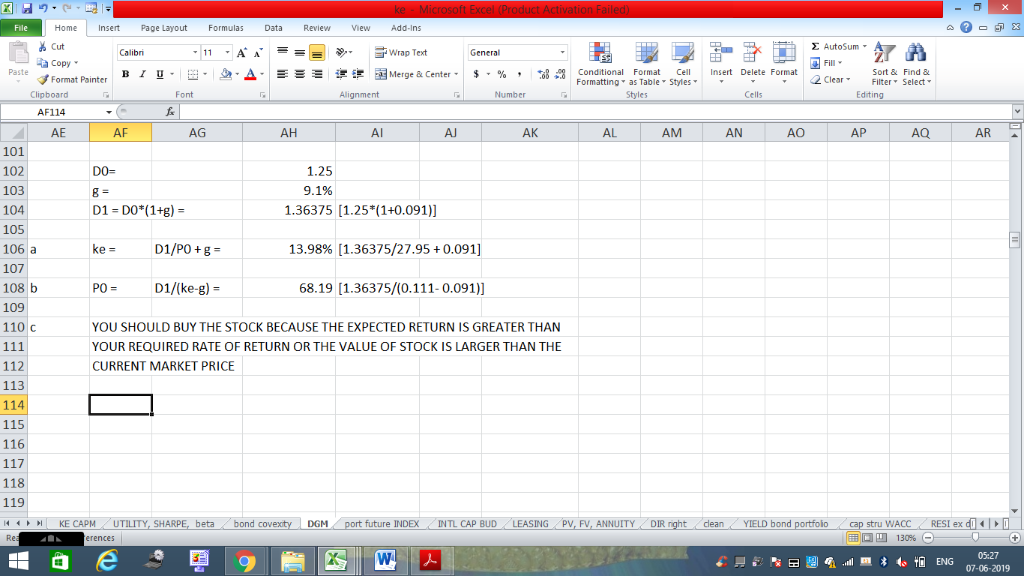In: Finance

# The common stock of NCP paid ​$1.25 in dividends last year. Dividends are expected to grow... The common stock of NCP paid ​$1.25 in dividends last year. Dividends are expected to grow at an annual rate of 9.10 percent for an indefinite number of years.

a. If​ NCP's current market price is ​$27.95 per​ share, the​ stock's expected rate of return is____%. ​(Round to two decimal​ places.) b. If your required rate of return is 11.1 ​percent, the value of the stock would be ​$ _____. ​(Round to the nearest​ cent.)

c. You should (buy or sell) the stock because the expected rate of return is ( less than or greater than) your required rate of return or the value of the stock is ( larger than or smaller than) the current market price.

## Solutions

##### Expert Solution## Related Solutions

​(Common stock​ valuation)  The common stock of NCP paid ​$1.32 in dividends last year. Dividends are expected to grow at an annual rate of 8.00 percent for an indefinite number of years. a. If your required rate of return is 10.50 percent​, what is the value of the stock for​ you? b. Should you make the​ investment? ##### Jacobson, Inc. paid$3.00 in dividends to common stockholderslast year. Dividends are expected to grow...
Jacobson, Inc. paid $3.00 in dividends to common stockholders last year. Dividends are expected to grow at sustainable growth rate for an indefinite number of years. Jacobson has a return on equity of 30% with dividend payout ratio of 60%.If Jacobson’s stock current market price is$33.60, what is the stock’s expected rate of return?If your required rate of return is 18 percent, what is the value of the stock for you?
The earnings, dividends, and common stock price of Shelby Inc. are expected to grow at 3% per year in the future. Shelby's common stock sells for $21.00 per share, its last dividend was$2.00, and the company will pay a dividend of $2.06 at the end of the current year. Using the discounted cash flow approach, what is its cost of equity? Round your answer to two decimal places. %....... If the firm's beta is 2.1, the risk-free rate is... ##### Mary's Bakery has common stock with a current price of$79 and paid dividends last year...
Mary's Bakery has common stock with a current price of $79 and paid dividends last year of$2.34. Common stock floatation costs are $12.65 per share and the growth rate on common stock is 7%. The firm's preferred stock sells for$109 with a $9.50 dividend per share and floatation costs of$6.25 per share.   use four decimals places in the calculation. Calculate the cost of preferred stock Calculate the cost of common stock Calculate the cost of retained earnings.
##### The future earnings, dividends, and common stock price of Callahan Technologies Inc. are expected to grow...
The future earnings, dividends, and common stock price of Callahan Technologies Inc. are expected to grow 6% per year. Callahan's common stock currently sells for $29.50 per share; its last dividend was$2.50; and it will pay a \$2.65 dividend at the end of the current year. Using the DCF approach, what is its cost of common equity? Do not round intermediate calculations. Round your answer to two decimal places.   % If the firm's beta is 0.7, the risk-free rate...